# 一、总览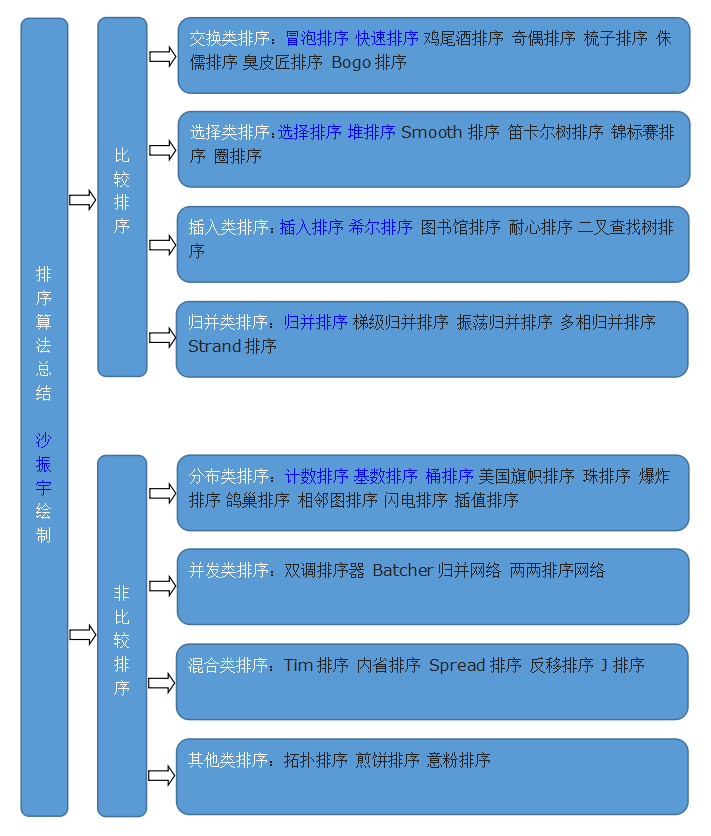# 二、常用排序算法

## 1、冒泡排序——交换类排序

### 1.2 源码

# 作者：沙振宇
# 冒泡排序
def bubbleSort(arr):
for i in range(1, len(arr)):
for j in range(0, len(arr)-i):
if arr[j] > arr[j+1]:
arr[j], arr[j + 1] = arr[j + 1], arr[j]
return arr

if __name__ == '__main__':
list = [1, 5, 8, 123, 22, 54, 7, 99, 300, 222]
print("List source is:", list)
result = bubbleSort(list)
print("List sort is:", result)


### 1.3 效果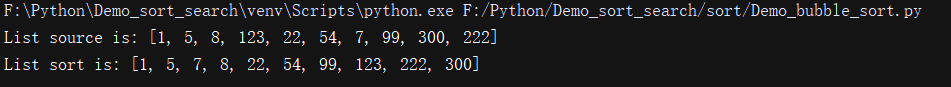## 2、快速排序——交换类排序

### 2.2 源码

# 作者：沙振宇
# 快速排序
def quickSort(arr, left=None, right=None):
left = 0 if not isinstance(left,(int, float)) else left
right = len(arr)-1 if not isinstance(right,(int, float)) else right
if left < right:
partitionIndex = partition(arr, left, right)
quickSort(arr, left, partitionIndex-1)
quickSort(arr, partitionIndex+1, right)
return arr

def partition(arr, left, right):
pivot = left
index = pivot+1
i = index
while  i <= right:
if arr[i] < arr[pivot]:
swap(arr, i, index)
index+=1
i+=1
swap(arr,pivot,index-1)
return index-1

def swap(arr, i, j):
arr[i], arr[j] = arr[j], arr[i]

if __name__ == '__main__':
list = [1, 5, 8, 123, 22, 54, 7, 99, 300, 222]
print("List source is:", list)
result = quickSort(list)
print("List sort is:", result)


### 2.3 快排简写

def quickSort2(array):
return array if len(array) <= 1 else quickSort2([lt for lt in array[1:] if lt < array]) + array[0:1] + quickSort2([ge for ge in array[1:] if ge >= array])


### 2.4 效果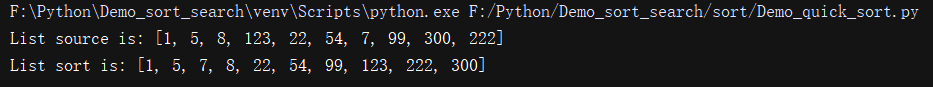## 3、选择排序——选择类排序

### 3.2 源码

# 作者：沙振宇
# 选择排序
def selectionSort(arr):
for i in range(len(arr) - 1):
# 记录最小数的索引
minIndex = i
for j in range(i + 1, len(arr)):
if arr[j] < arr[minIndex]:
minIndex = j
# i 不是最小数时，将 i 和最小数进行交换
if i != minIndex:
arr[i], arr[minIndex] = arr[minIndex], arr[i]
return arr

if __name__ == '__main__':
list = [1, 5, 8, 123, 22, 54, 7, 99, 300, 222]
print("List source is:", list)
result = selectionSort(list)
print("List sort is:", result)


### 3.3 效果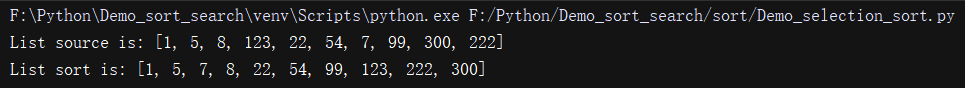## 4、堆排序——选择类排序

### 4.1 简介

1、大顶堆（大根堆）：每个节点的值都大于或等于其子节点的值，在堆排序算法中用于升序排列；
2、小顶堆（小根堆）：每个节点的值都小于或等于其子节点的值，在堆排序算法中用于降序排列；

### 4.2 源码

# 作者：沙振宇
# 堆排序
def buildMaxHeap(arr):
import math
for i in range(math.floor(len(arr)/2),-1,-1):
heapify(arr,i)

def heapify(arr, i):
left = 2*i+1
right = 2*i+2
largest = i
if left < arrLen and arr[left] > arr[largest]:
largest = left
if right < arrLen and arr[right] > arr[largest]:
largest = right
if largest != i:
swap(arr, i, largest)
heapify(arr, largest)

def swap(arr, i, j):
arr[i], arr[j] = arr[j], arr[i]

def heapSort(arr):
global arrLen
arrLen = len(arr)
buildMaxHeap(arr)
for i in range(len(arr)-1,0,-1):
swap(arr,0,i)
arrLen -=1
heapify(arr, 0)
return arr

if __name__ == '__main__':
list = [1, 5, 8, 123, 22, 54, 7, 99, 300, 222]
print("List source is:", list)
result = heapSort(list)
print("List sort is:", result)


### 4.3 效果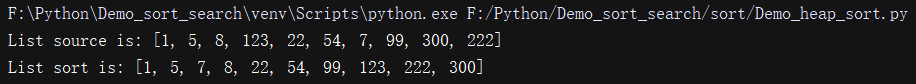## 5、插入排序——插入类排序

### 5.2 源码

# 作者：沙振宇
# 插入排序
def insertionSort(arr):
for i in range(len(arr)):
preIndex = i-1
current = arr[i]
while preIndex >= 0 and arr[preIndex] > current:
arr[preIndex+1] = arr[preIndex]
preIndex-=1
arr[preIndex+1] = current
return arr

if __name__ == '__main__':
list = [1, 5, 8, 123, 22, 54, 7, 99, 300, 222]
print("List source is:", list)
result = insertionSort(list)
print("List sort is:", result)


### 5.3 效果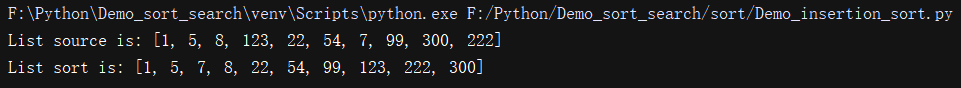## 6、希尔排序——插入类排序

### 6.1 简介

1、插入排序在对几乎已经排好序的数据操作时，效率高，即可以达到线性排序的效率；
2、但插入排序一般来说是低效的，因为插入排序每次只能将数据移动一位；

### 6.2 源码

# 作者：沙振宇
# 希尔排序
def shellSort(arr):
import math
gap=1
while(gap < len(arr)/3):
gap = gap*3+1
while gap > 0:
for i in range(gap,len(arr)):
temp = arr[i]
j = i-gap
while j >=0 and arr[j] > temp:
arr[j+gap]=arr[j]
j-=gap
arr[j+gap] = temp
gap = math.floor(gap/3)
return arr

if __name__ == '__main__':
list = [1, 5, 8, 123, 22, 54, 7, 99, 300, 222]
print("List source is:", list)
result = shellSort(list)
print("List sort is:", result)


### 6.3 效果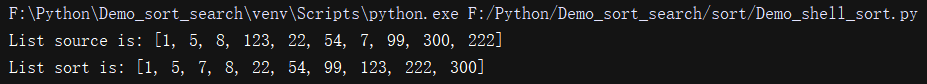## 7、归并排序——归并类排序

### 7.1 简介

1、自上而下的递归（所有递归的方法都可以用迭代重写，所以就有了第 2 种方法）；
2、自下而上的迭代；

### 7.2 源码

# 作者：沙振宇
# 归并排序
def mergeSort(arr):
import math
if(len(arr)<2):
return arr
middle = math.floor(len(arr)/2)
left, right = arr[0:middle], arr[middle:]
return merge(mergeSort(left), mergeSort(right))

def merge(left,right):
result = []
while left and right:
if left <= right:
result.append(left.pop(0))
else:
result.append(right.pop(0))
while left:
result.append(left.pop(0))
while right:
result.append(right.pop(0))
return result

if __name__ == '__main__':
list = [1, 5, 8, 123, 22, 54, 7, 99, 300, 222]
print("List source is:", list)
result = mergeSort(list)
print("List sort is:", result)


### 7.3 效果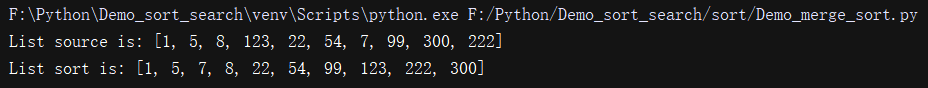## 8、计数排序——分布类排序

### 8.2 源码

# 作者：沙振宇
# 计数排序
def countingSort(arr, maxValue):
bucketLen = maxValue+1
bucket = *bucketLen
sortedIndex =0
arrLen = len(arr)
for i in range(arrLen):
if not bucket[arr[i]]:
bucket[arr[i]]=0
bucket[arr[i]]+=1
for j in range(bucketLen):
while bucket[j]>0:
arr[sortedIndex] = j
sortedIndex+=1
bucket[j]-=1
return arr

if __name__ == '__main__':
list = [1, 5, 8, 123, 22, 54, 7, 99, 300, 222]
print("List source is:", list)
result = countingSort(list,max(list))
print("List sort is:", result)


### 8.3 效果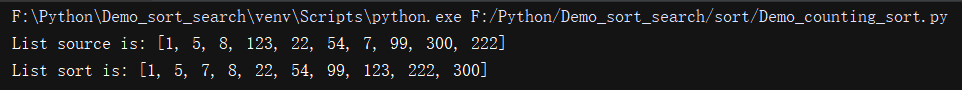## 9、基数排序——分布类排序

### 9.2 源码

# 作者：沙振宇
# 基数排序
"""基数排序"""
i = 0 # 记录当前正在排拿一位，最低位为1
max_num = max(arr)  # 最大值
j = len(str(max_num))  # 记录最大值的位数
while i < j:
bucket_list =[[] for _ in range(10)] #初始化桶数组
for x in arr:
bucket_list[int(x / (10**i)) % 10].append(x) # 找到位置放入桶数组
arr.clear()
for x in bucket_list:   # 放回原序列
for y in x:
arr.append(y)
i += 1
return arr

if __name__ == '__main__':
list = [1, 5, 8, 123, 22, 54, 7, 99, 300, 222]
print("List source is:", list)
print("List sort is:", result)


### 9.3 效果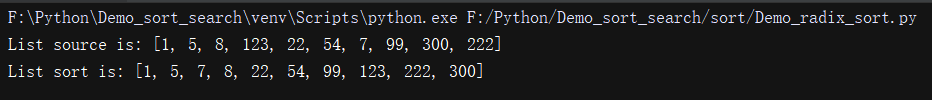## 10、桶排序——分布类排序

### 10.1 简介

1、在额外空间充足的情况下，尽量增大桶的数量
2、使用的映射函数能够将输入的 N 个数据均匀的分配到 K 个桶中

### 10.2 源码

# 作者：沙振宇
# 桶排序
def bucketSort(arr):
# 选择一个最大的数
max_num = max(arr)
# 创建一个元素全是0的列表, 当做桶
bucket =  * (max_num + 1)
# 把所有元素放入桶中, 即把对应元素个数加一
for i in arr:
bucket[i] += 1
# 存储排序好的元素
sort_nums = []
# 取出桶中的元素
for j in range(len(bucket)):
if bucket[j] != 0:
for y in range(bucket[j]):
sort_nums.append(j)
return sort_nums

if __name__ == '__main__':
list = [1, 5, 8, 123, 22, 54, 7, 99, 300, 222]
print("List source is:", list)
result = bucketSort(list)
print("List sort is:", result)


### 10.3 效果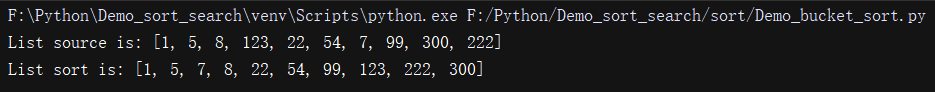# 三、Github源码分享

https://github.com/ShaShiDiZhuanLan/Demo_SortSearch_Python

05-2475508-212万+
04-262万+
06-214973
03-217万+
07-234万+
07-041万+
05-281万+
03-12138
07-249422